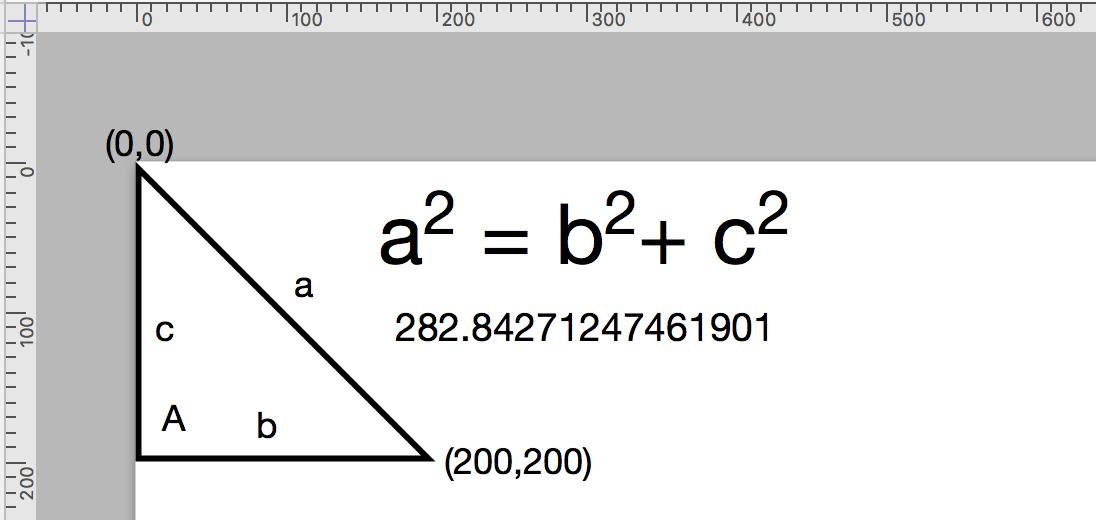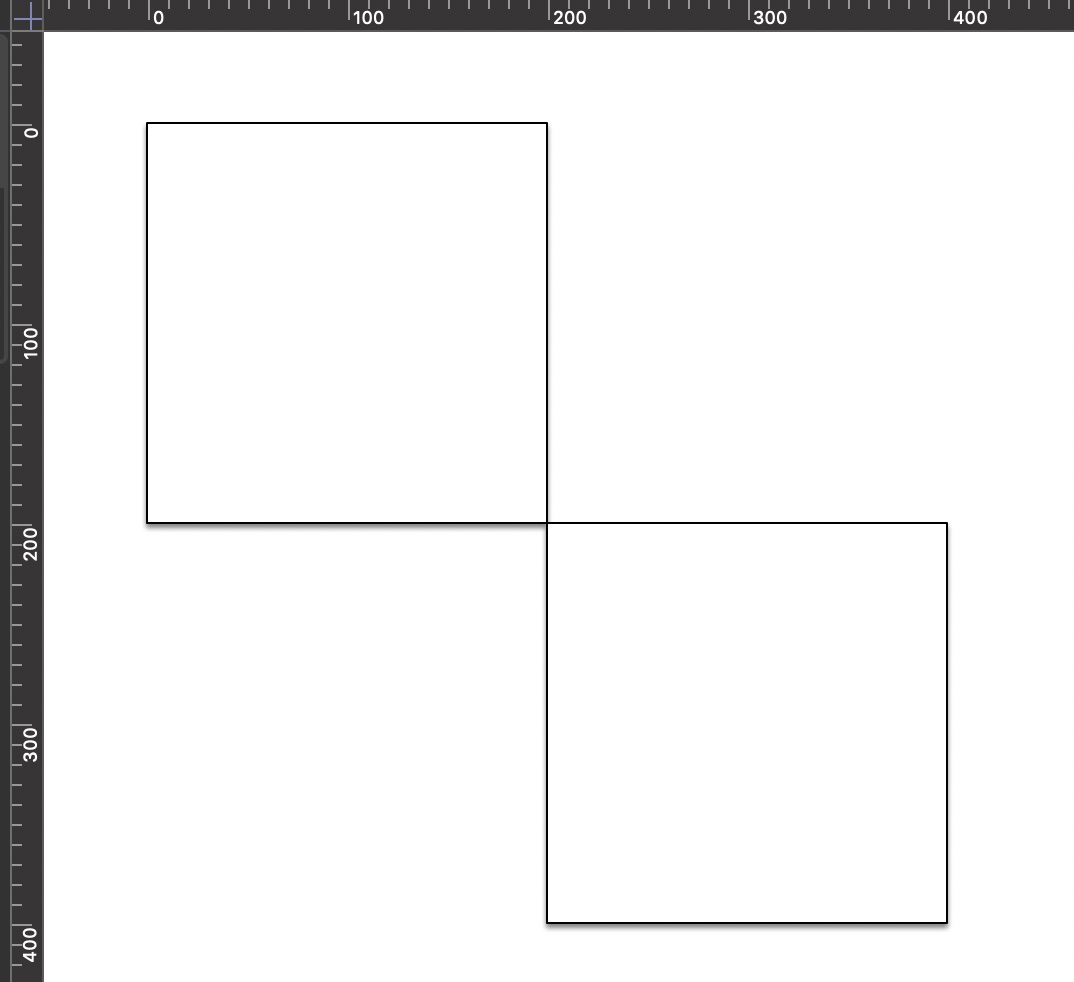×

# Point

A Point is a class describing the position of a graphic. It has two elements, the horizontal offset (in points) and the vertical offset (in points) of the object from the canvas origin. A Point is also the first component of a Rect, which describes both the location (Point) and dimensions (Size) of a graphic.

## Creating a Point

An instance of the Point class is created by preceding the class name with the new item constructor, and following the class name with the horizontal offset (in points), and vertical offset (in points) of the object, enclosed in a pair of parens (). The result is a new instance of the Point class:

• new Point(horizontalOffset, verticalOffset) (Point) • Returns a new Point with the specified coordinates.

```Create a New Point

`var pt = new Point(72, 90)`
`//--> [object Point: (72.0, 90.0)]`
```

## Class Properties

By default, the Point class has three intrinsic properties for creating a defined point.

• unitX (Point r/o) • Returns a Point with coordinates (1, 0)

• unitY (Point r/o) • Returns a Point with coordinates (0, 1)

• zero (Point r/o) • Returns the Point (0, 0), the origin.

```Point Properties

`const ptX = Point.unitX`
`//--> [object Point: (1.0, 0.0)]`
`const ptY = Point.unitY`
`//--> [object Point: (0.0, 1.0)]`
`const pt0 = Point.zero`
`//--> [object Point: (0.0, 0.0)]`
```

## Instance Properties

An instance of the Point class has five properties which can be accessed by scripts: length, negative, normalized, x, and y

• length (Number r/o) • Returns the distance between the receiver and the origin.

```The Length of a Point

`const pt = new Point(200, 200)`
`pt.length`
`//--> 282.842712474619`
```

The value of the length property is the Point’s distance from the canvas origin. In essence, this value is length of the hypotenuse of the right triangle formed by the horizontal and vertical offsets of the point. It is determined using the Pythagorean theorem.• negative (Number r/o) • Returns the component-wise negative of the receiver.

```The Negative of a Point

`const pt = new Point(100, 200)`
`pt.negative`
`//--> [object Point: (-100.0, -200.0)]`
```
• normalized (Number r/o) • For a non-zero point, returns a point with a distance of one from the origin, but in the same direction as the original. For the zero point, this returns the receiver.

```Normalized Point

`const pt = new Point(100, 200)`
`pt.normalized`
`//--> [object Point: (0.447213595499958, 0.894427190999916)]`
```
• x (Number r/o) • The horizontal axis coordinate of the Point.

```The Horiontal Component of a Point

`const pt = new Point(100, 200)`
`pt.x`
`//--> 100`
```
• y (Number r/o) • The verticql axis coordinate of the Point.

```The Vertical Component of a Point

`const pt = new Point(100, 200)`
`pt.y`
`//--> 200`
```

## Instance Functions

There are three functions you can use to derive a new point based upon an instance of a point, and two functions for deriving a measurement from a point instance.

• add(point: Point) → (Point) • Returns a new Point that is the component-wise sum of the receiver and the argument Point

• subtract(point: Point) → (Point) • Returns a new Point that is the component-wise difference of the receiver and the argument Point

• scale(factor: Number) → (Point) • Returns a new Point where each component is scaled by the given factor.

• distanceTo(point: Point) → (Number) • Returns the distance between the receiver and the given Point

• dot(point: Point) → (Number) • Returns the dot product between the receiver and the given Point.

Here’s an example script that uses the add() instance function with the duplicateTo() graphic function to create a diagonal row of squares:

omnigraffle://localhost/omnijs-run?script=try%7Bvar%20cnvs%20%3D%20document%2Ewindows%5B0%5D%2Eselection%2Ecanvas%0Avar%20side%20%3D%20100%0Avar%20pt%20%3D%20new%20Point%28side%2Cside%29%0Avar%20shape%20%3D%20cnvs%2EaddShape%28%27Rectangle%27%2Cnew%20Rect%280%2C%200%2C%20side%2C%20side%29%29%0Afor%28i%20%3D%200%3B%20i%20%3C%203%3B%20i%2B%2B%29%7B%0A%09shape%20%3D%20shape%2EduplicateTo%28shape%2Egeometry%2Eorigin%2Eadd%28pt%29%29%0A%7D%7Dcatch%28err%29%7Bconsole%2Elog%28err%29%7D
```Adding Points

`var cnvs = document.windows.selection.canvas`
`var side = 100`
`var pt = new Point(side,side)`
`var shape = cnvs.addShape('Rectangle',new Rect(0, 0, side, side))`
`for(i = 0; i < 3; i++){`
`	shape = shape.duplicateTo(shape.geometry.origin.add(pt))`
`}`
```Using the scale function to diagonally duplicate a shape:

omnigraffle://localhost/omnijs-run?script=try%7Bvar%20cnvs%20%3D%20document%2Ewindows%5B0%5D%2Eselection%2Ecanvas%0Avar%20shape%20%3D%20cnvs%2EaddShape%28%27Rectangle%27%2Cnew%20Rect%280%2C%200%2C%20200%2C%20200%29%29%0Ashape%2EduplicateTo%28shape%2Egeometry%2Ecenter%2Escale%282%29%29%7Dcatch%28err%29%7Bconsole%2Elog%28err%29%7D
```Using Scale Function

`var cnvs = document.windows.selection.canvas`
`var shape = cnvs.addShape('Rectangle',new Rect(0, 0, 200, 200))`
`shape.duplicateTo(shape.geometry.center.scale(2))`
```